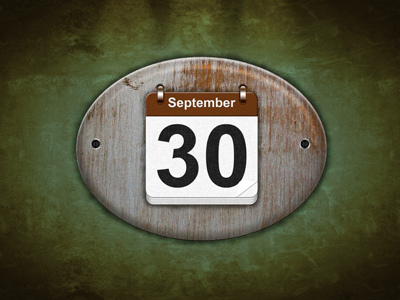30 is a multiple of 5, 6 and 15.

# Multiples 4

This Math quiz is called 'Multiples 4' and it has been written by teachers to help you if you are studying the subject at elementary school. Playing educational quizzes is an enjoyable way to learn if you are in the 3rd, 4th or 5th grade - aged 8 to 11.

It costs only \$12.50 per month to play this quiz and over 3,500 others that help you with your school work. You can subscribe on the page at Join Us

As a reminder, a 'multiple' is a number that can be divided exactly by another given number, e.g. 8 is a multiple of 2 because 2 divides 8 exactly four times. By the way, 5.4 is a multiple of 1.8 because 1.8 divides 5.4 exactly three times.

In this quiz we shall only be dealing with whole numbers BUT it's a good idea to keep a lookout for multiples when you work with decimals. The times tables that you have learned will help you in this quiz.

1.
Which of the four choices is a multiple of ALL the numbers given?
4, 8, 16, 32
64
36
68
126
36 and 68 are only divisible by 4, and 126 isn't divisible by any of them
2.
Which of the four choices is a multiple of ALL the numbers given?
5, 6, 15
30
75
36
45
75 isn't divisible by 6, 36 is only divisible by 6 and 45 isn't divisible by 6
3.
Which of the four choices is a multiple of ALL the numbers given?
11, 12
121
132
144
111
121 isn't divisible by 12, 144 isn't divisible by 11 and 111 isn't divisible by either of them
4.
Which of the four choices is a multiple of ALL the numbers given?
7, 6, 14
82
48
42
86
82 isn't divisible by any, 48 is only divisible by 6 and 86 isn't divisible by any
5.
Which of the four choices is a multiple of ALL the numbers given?
6, 11, 12
132
66
72
121
A good strategy is as follows: find a likely multiple and check to see if one of the given numbers DOESN'T divide it. If you can't find one, then that's your multiple. If you do find one, then that's NOT your multiple, e.g. 12 doesn't divide 66
6.
Which of the four choices is a multiple of ALL the numbers given?
3, 9, 11
33
27
99
66
33 isn't divisible by 9, 27 isn't divisible by 11 and 66 isn't divisible by 9
7.
Which of the four choices is a multiple of ALL the numbers given?
8, 9, 12
96
72
84
74
96 isn't divisible by 9, 84 is only divisible by 12 and 74 isn't divisible by any of them
8.
Which of the four choices is a multiple of ALL the numbers given?
6, 7, 8
252
42
48
336
A good strategy is as follows: find a likely multiple and check to see if one of the given numbers DOESN'T divide it. If you can't find one, then that's your multiple. If you do find one, then that's NOT your multiple, e.g. 8 doesn't divide 42. TIP! If you see really big likely multiples, try multiplying the given numbers to see if they can form them: 6 × 7 × 8 = 336
9.
Which of the four choices is a multiple of ALL the numbers given?
6, 9, 18
52
27
48
36
52 isn't divisible by any of them, 27 is only divisible by 9 and 48 is only divisible by 6
10.
Which of the four choices is a multiple of ALL the numbers given?
15, 25, 30
300
230
375
525
230 isn't divisible by any of them. 375 and 525 aren't divisible by 30
Author:  Frank Evans